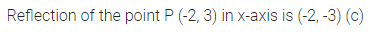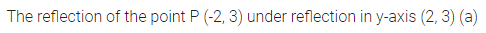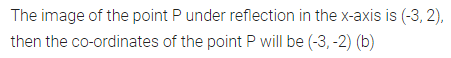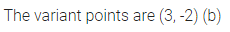# ML Aggarwal Class 10 Solutions for ICSE Maths Chapter 10 Reflection MCQS

## ML Aggarwal Class 10 Solutions for ICSE Maths Chapter 10 Reflection MCQS

ML Aggarwal Class 10 Solutions for ICSE Maths Chapter 10 Reflection MCQS

Choose the correct answer from the given four options (1 to 7):

Question 1.
The reflection of the point P (-2, 3) in the x-axis is
(a) (2, 3)
(b) (2, -3)
(c) (-2, -3)
(d) (-2, 3)
Solution:Question 2.
The reflection of the point P (-2, 3) in the y- axis is
(a) (2, 3)
(b) (2, -3)
(c) (-2, -3)
(d) (0, 3)
Solution:Question 3.
If the image of the point P under reflection in the x-axis is (-3, 2), then the coordinates of the point P are
(a) (3, 2)
(b) (-3, -2)
(c) (3, -2)
(d) (-3, 0)
Solution:Question 4.
The reflection of the point P (1, -2) in the line y = -1 is
(a) ( -3, -2)
(b) (1, – 4)
(c) (1 , 4)
(d) (1, 0)
Solution:Question 5.
The reflection of the point A (4, -1) in the line x = 2 is
(a) (0, -1)
(b) (8, -1)
(c) (0, 1)
(d) none of these
Solution:Question 6.
The reflection of the point (-3, 0) in the origin is the point
(a) (0, -3)
(b) (0, 3)
(c) (3, 0)
(d) none of these
Solution:Question 7.
Which of the following points is invariant with respect to the line y = -2 ?
(a) (3, 2)
(b) (3, -2)
(c) (2, 3)
(d) (-2, 3)
Solution:ML Aggarwal Class 10 Solutions for ICSE Maths